# SCERT Maharashtra Question Bank solutions for 10th Standard SSC Science and Technology 1 Maharashtra State Board 2021 chapter 6 - Refraction of light [Latest edition]

#### Chapters## Chapter 6: Refraction of light

Choose the correct option.Find the correlation.Answer in one sentence.Right or Wrong sentenceWrite an ExplanationWrite scientific reasonsSolve the following QuestionsAnswer the Following
Choose the correct option.

### SCERT Maharashtra Question Bank solutions for 10th Standard SSC Science and Technology 1 Maharashtra State Board 2021 Chapter 6 Refraction of light Choose the correct option.

#### Choose the correct option.

Choose the correct option. | Q 1

Due to _______ pencil looks bent in water, in the given experiment.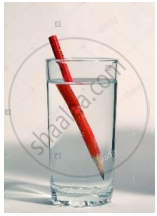• refraction of light

• dispersion of light

• internal reflection of light

• reflection of light

Choose the correct option. | Q 2

In the following diagram if ∠ i = 40°, then ∠ e = _______?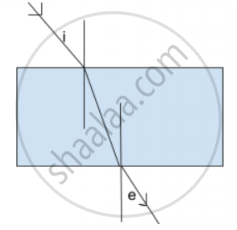• 50°

• 40°

• 60°

• 90°

Choose the correct option. | Q 3

A ray of light strikes the glass slab at an angle 40° with the surface of the slab. Then the angle of incidence will be _______°.

• 50

• 40

• 60

• 90

Choose the correct option. | Q 4

We can see the Sun even when it is little below the horizon because of ______.

•  Reflection of light

• Refraction of light

• Dispersion of light

• Absorption of light

Choose the correct option. | Q 5

_______ is the unit of refractive index.

• cm

• m

• degree

• refractive index has no unit

Choose the correct option. | Q 6

n = _______. This law is also called as Snell’s law.

• (sin "r")/(sin "i")

• (sin "r")/(sin "e")

• (sin "e")/(sin "r")

• (sin "i")/(sin "r")

Choose the correct option. | Q 7

Lights of different colours are used as signal for safety transport. From these, the wavelength of red light is _______ nm.

• 400

• 500

• 600

• 700

Choose the correct option. | Q 8

If the refractive index of air with respect to glass is 2/3. What is the refractive index of glass with respect to air?

• 2/3

• 3/2

• 1/3

• 1/2

Choose the correct option. | Q 9

The process of separation of light into its component colours while passing through a medium is called _______.

• reflection of light

• refraction of light

• dispersion of light

• absorption of light

Choose the correct option. | Q 10

Light changes its direction when going from one transparent medium to another transparent medium. This is called _______.

• reflection of light

• refraction of light

• dispersion of light

• absorption of light

Find the correlation.

### SCERT Maharashtra Question Bank solutions for 10th Standard SSC Science and Technology 1 Maharashtra State Board 2021 Chapter 6 Refraction of light Find the correlation.

#### Find the correlation.

Find the correlation. | Q 1

2n1 : Refractive index of medium 2 with respect to medium 1 : : 1n2 : _______

Find the correlation. | Q 2

Refractive index of air : 1.0003 : : refractive index of water : _______

### SCERT Maharashtra Question Bank solutions for 10th Standard SSC Science and Technology 1 Maharashtra State Board 2021 Chapter 6 Refraction of light Answer in one sentence.

Answer in one sentence. | Q 1

Identify the wrong figure from the following.

•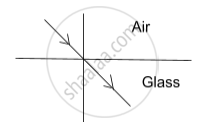•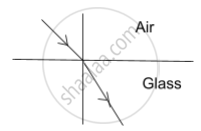Right or Wrong sentence

### SCERT Maharashtra Question Bank solutions for 10th Standard SSC Science and Technology 1 Maharashtra State Board 2021 Chapter 6 Refraction of light Right or Wrong sentence

Right or Wrong sentence | Q 1

The incident rays and refracting rays are on opposite sides of the normal.

• Right

• Wrong

Right or Wrong sentence | Q 2

Purple has the lowest refractive index.

• Right

• Wrong

Right or Wrong sentence | Q 3

The speed of light varies in different media.

• Right

• Wrong

Write an Explanation

### SCERT Maharashtra Question Bank solutions for 10th Standard SSC Science and Technology 1 Maharashtra State Board 2021 Chapter 6 Refraction of light Write an Explanation

Write an Explanation | Q 1

Write an Explanation.

Critical angle

Write scientific reasons

### SCERT Maharashtra Question Bank solutions for 10th Standard SSC Science and Technology 1 Maharashtra State Board 2021 Chapter 6 Refraction of light Write scientific reasons

Write scientific reasons | Q 1

Write scientific reason.

The sun appears on the western horizon for some time after sunset.

Write scientific reasons | Q 2

Write scientific reason.

The bottom of a pond appears raised.

Write scientific reasons | Q 3

Write scientific reason.

Stars twinkle but we do not see the twinkling of planets.

Write scientific reasons | Q 4

Write scientific reason.

The coin disappears in a vessel as it is seen from a specific location. But as soon as the water is poured into the vessel to a certain level, the coin appears.

Write scientific reasons | Q 5

Write scientific reason.

A pencil appears to be broken near the surface of water.

Solve the following Questions

### SCERT Maharashtra Question Bank solutions for 10th Standard SSC Science and Technology 1 Maharashtra State Board 2021 Chapter 6 Refraction of light Solve the following Questions

Solve the following Questions | Q 1

If the absolute refractive index of water is 1.36, find the speed of light in water. (Speed of light in vacuum = 3 × 108 m/s.)

Solve the following Questions | Q 2

If the absolute refractive index of glass and water are 3/2 and 4/3 respectively, what is the refractive index of glass with respect to water?

Solve the following Questions | Q 3

Solve the following example.
Speed in first medium, v1 = 3 × 108 m/s
Speed in second medium, v2 = 2 × 108 m/s
Then 2n1 = ?

Solve the following Questions | Q 4

Draw a neat and labelled diagram for dispersion of light.

Solve the following Questions | Q 5

Observe the given figure and name the following.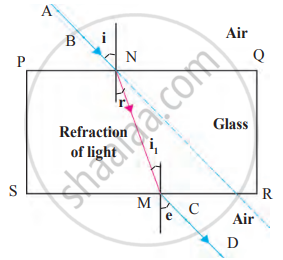Ray AB Ray NM Ray MD ∠ r
Solve the following Questions | Q 6

What is refraction of light?

Solve the following Questions | Q 8

State the laws of refraction of light.

Solve the following Questions | Q 9

If in a medium, the speed of light is 1.5 × 108 m/s how much will the absolute refractive index of that medium be?

### SCERT Maharashtra Question Bank solutions for 10th Standard SSC Science and Technology 1 Maharashtra State Board 2021 Chapter 6 Refraction of light Answer the Following

Answer the Following | Q 1

Rainbow is a beautiful natural phenomenon. It is the combined effect of a natural three processes together produced by light. Write it into the circle.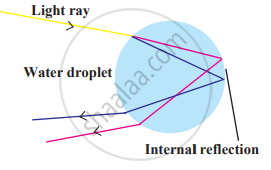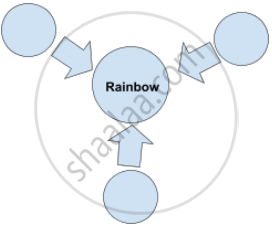Answer the Following | Q 2.1

Observe the given figure and write appropriate phenomenon of light in the box.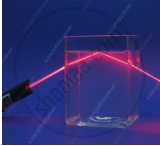Answer the Following | Q 2.2

Observe the given figure and write appropriate phenomenon of light in the box.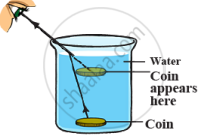Answer the Following | Q 2.3

Observe the given figure and write appropriate phenomenon of light in the box.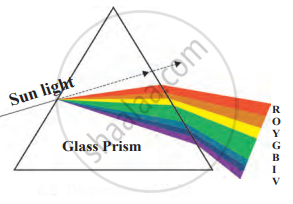Answer the Following | Q 3

Observe the given figure and answer the following questions.1. Which colour light rays bend most?
2. Which colour light rays bend least.
3. What is the wavelength of violet light rays?

## Chapter 6: Refraction of light

Choose the correct option.Find the correlation.Answer in one sentence.Right or Wrong sentenceWrite an ExplanationWrite scientific reasonsSolve the following QuestionsAnswer the Following## SCERT Maharashtra Question Bank solutions for 10th Standard SSC Science and Technology 1 Maharashtra State Board 2021 chapter 6 - Refraction of light

SCERT Maharashtra Question Bank solutions for 10th Standard SSC Science and Technology 1 Maharashtra State Board 2021 chapter 6 (Refraction of light) include all questions with solution and detail explanation. This will clear students doubts about any question and improve application skills while preparing for board exams. The detailed, step-by-step solutions will help you understand the concepts better and clear your confusions, if any. Shaalaa.com has the Maharashtra State Board 10th Standard SSC Science and Technology 1 Maharashtra State Board 2021 solutions in a manner that help students grasp basic concepts better and faster.

Further, we at Shaalaa.com provide such solutions so that students can prepare for written exams. SCERT Maharashtra Question Bank textbook solutions can be a core help for self-study and acts as a perfect self-help guidance for students.

Concepts covered in 10th Standard SSC Science and Technology 1 Maharashtra State Board 2021 chapter 6 Refraction of light are Refraction of Light, Law of Refraction of Light, Refractive Index, Application of Atmospheric Refraction, Concept of Prism, Dispersion of Light Through Prism and Formation of Spectrum, Partial Internal Reflection, Total Internal Reflection, Refraction of Light Through a Rectangular Glass Slab.

Using SCERT Maharashtra Question Bank 10th Standard [इयत्ता १० वी] solutions Refraction of light exercise by students are an easy way to prepare for the exams, as they involve solutions arranged chapter-wise also page wise. The questions involved in SCERT Maharashtra Question Bank Solutions are important questions that can be asked in the final exam. Maximum students of Maharashtra State Board 10th Standard [इयत्ता १० वी] prefer SCERT Maharashtra Question Bank Textbook Solutions to score more in exam.

Get the free view of chapter 6 Refraction of light 10th Standard [इयत्ता १० वी] extra questions for 10th Standard SSC Science and Technology 1 Maharashtra State Board 2021 and can use Shaalaa.com to keep it handy for your exam preparation